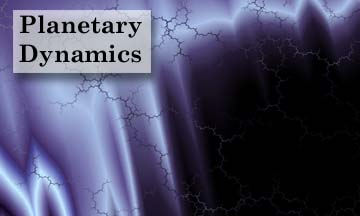Introduction

By planetary dynamics, we mean the study of the orbits of various celestial bodies and the dynamics of orientation of spinning bodies. The content is not strictly limited to celestial bodies of planetary size. Natural and artificial satellite dynamics and orbits can also be considered under this classification.

For example, the a body's spin axis (such as the Earth or of a spin stabilized satellite) is not stationary relative to the stars. In such cases, the body is said to be undergoing precession and nutation. In the case of a planet, the spin axis may not be stationary relative to the crust of the planet's surface either. In this case the physical process is called polar wander.

In terms of celestial mechanics, the primary source for the forces acting on an orbiting body is due to the gravitational potential of the central body. The solution of orbits is an old problem, but one that contains considerable beauty and theoretical depth. Some of mankind's greatest mathematical minds have contributed to the solution of these problems.

Resources

• Hard Copy:

• Celestial Mechanics, Satellite Geodesy and Spherical Astronomy Texts

• Baker R. M. L. & M. W. Makemson, An Introduction to Astrodynamics, Academic Press, New York, 1960.
• Brouwer, D. & G. M. Clemence, Methods of Celestial Mechanics, Academic Press, New York, 1961.
• Chauvenet, W., A Manual of Spherical and Practical Astronomy, 2 vols., Dover reprint, New York, 1891.
• Escobal, P. R., Methods of Orbit Determination, Krieger Publ Co. (reprint of Wiley & Sons), Malabar, FL, 1965.
• Kaula, W. M., Theory of Satellite Geodesy, Dover reprint, New York, 1966.
• Kaplan, J. M. A., Fundamentals of Celestial Mechanics, MacMillan Co., New York, 1962.
• McCusky, S. W., Introduction to Celestial Mechanics, Addison Wesley, Reading, MA, 1963.
• Montenbruck, O. & E. Gill, Satellite Orbits, Springer Verlag, Berlin, 2000.
• Moulton, F. R., An Introduction to Celestial Mechanics, Dover reprint, New York, 1914.
• Mueller, I. I., Spherical Astronomy as Applied to Geodesy, F. Ungar, New York, 1969.
• Newcomb, S., A Compendium of Spherical Astronomy, Dover reprint, New York, 1906.
• Schneider, M., Himmelsmechanik, 4 vols. in German.
• Vol. 1: Grundlagen & Determinierung, BI Wissenschaftsverlag, Mannheim, 1992. 657pp.
• Vol. 2: Systemmodelle, BI Wissenschaftsverlag, Mannheim, 1993. 720pp.
• Vol. 3: Gravitationstheorie, Spektrum Akademischer Verlag, Heidelberg, 1996. 871pp.
• Vol. 4: Theorie der Satellitenbewegung, Bahnbestimmung, Spektrum Akademischer Verlag, Heidelberg, 1999. 1143pp.
• Smart, Spherical Astronomy, Cambridge University Press, Cambridge, 1977 (more recent editions exist).
• Vallado, D. A., Fundamentals of Astrodynamics and Applications, McGraw-Hill, New York, 1997.

• Planetary Rotation Texts

• Bostrom, R. C., Tectonic Consequences of the Earth's Rotation, Oxford U. Press, Oxford, 1999.
• Lambeck, K., The Earth's Variable Rotation: Geophysical Causes and Consequences, Cambridge U. Press, Cambridge, 1980.
• Lambeck, K., Geophysical Geodesy, Oxford U. Press, Oxford, 1988.
• Moritz, H. & I. I. Mueller, Earth Rotation Theory and Observation, F. Ungar, New York, 1987.
• Munk, W. M. & G. F. K. MacDonald, The Rotation of the Earth, Cambridge U. Press, Cambridge, 1960.

• Dynamics of Rigid and Semi-Rigid Bodies - Theoretical Texts

• MacMillan, W. D., Dynamics of Rigid Bodies, Dover reprint, 1936.
• Routh, E. J., Dynamics of a System of Rigid Bodies, 2 Vols., Dover reprint, New York, 1905.

• Internet:

Planetary Orbital Mechanics

• Local:

• Contents of McCusky's Introduction to Celestial Mechanics:

 Fundamental Dynamics Introduction Kinematics of Curvilinear Motion Areal Velocity Dynamics of Curvilinear Motion Potential Due to a Sphere Systems of Particles Central Force Motion The Law of Areas Linear and Angular Velocities Integrals of Angular Momentum and Energy The Equation of the Orbit The Inverse Square Force From Orbit to Force Law The Two-Body Problem Motion of the Center of Mass Relative Motion The Integral of Areas Elements of the Orbit from Initial Position and Velocity Properties of the Motion The Constant of Gravitation Kepler's Equation Position in the Elliptical Orbit Position in a Parabolic Orbit Position in a Hyperbolic Orbit Position in Near-Parabolic Orbits Position on the Celestial Sphere Computation of Orbits The Radius Vector is Known Laplace's Method Gauss's Method The Three- and n-Body Problem Motion of the Center of Mass The Angular Momentum, or Areal Velocity, Integrals The Integral of Energy Summary of Properties: n-Body Problem Equations of Relative Motion Stationary Solutions of the Three Body Problem The Restricted Three Body Problem Stabillity of Motion Near the Lagrangian Points The Theory of Perturbations Variation of Parameters Properties of Langrange Brackets Evaluation of Lagrange's Brackets Solution of Perturbation Equations Expansion of Perturbation Functions The Earth-Moon System Potential Due to an Oblate Spheroid Perturbations Due to an Oblate Planet Perturbations Due to Atmospheric Drag

• Contents of Schneider's Himmelsmechanik, Vol. 4.

 German English Theorie der Satellitenbewegung A. Kräfte und Drehmomente im Flugbereich B. Intermediäre Lösungen zur Bahnbewegung C. Intermediäre Lösungen zur Satellitendynamik D. Theorie der Bahnbewegung E. Satellitendynamik F. Natürliche Monde und Ringsysteme Bahn- und Parameterbestimmung A. Reduktion der Observablen B. Vorläufige Bahnbestimmung C. Definitive Bahnbestimmung D. Parameterbestimmung Schlußbetrachtung Anhänge A. Rekursive Berechnung der Neigungsfunktionen und ihrer Ableitungen B. Rekursive Berechnung der Exzentrizitätsfunktionen und ihrer Ableitungen Theory of the satellite-motion A. powers and (turn)-moments into the flight-area B. Intermediate solutions in Orbit Estimation C. Intermediate solutions to satellite dynamics D. Theory of Orbits E. Satellite Dynamics F. Natural moons and ring-systems Orbit and parameter-determination A. Reduction of the Observables B. Prediction of Orbits C. Definitive Orbit-determination D. parameter-determination Concluding Remarks A. Recursive calculation of the inclination functions and their derivation B. Recursive calculation of the eccentricity functions and their derivation